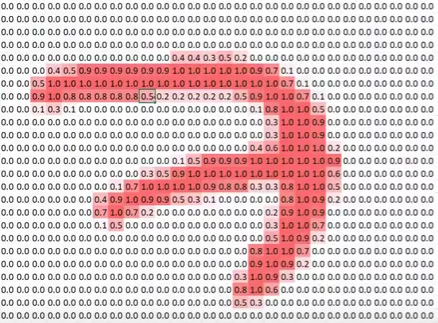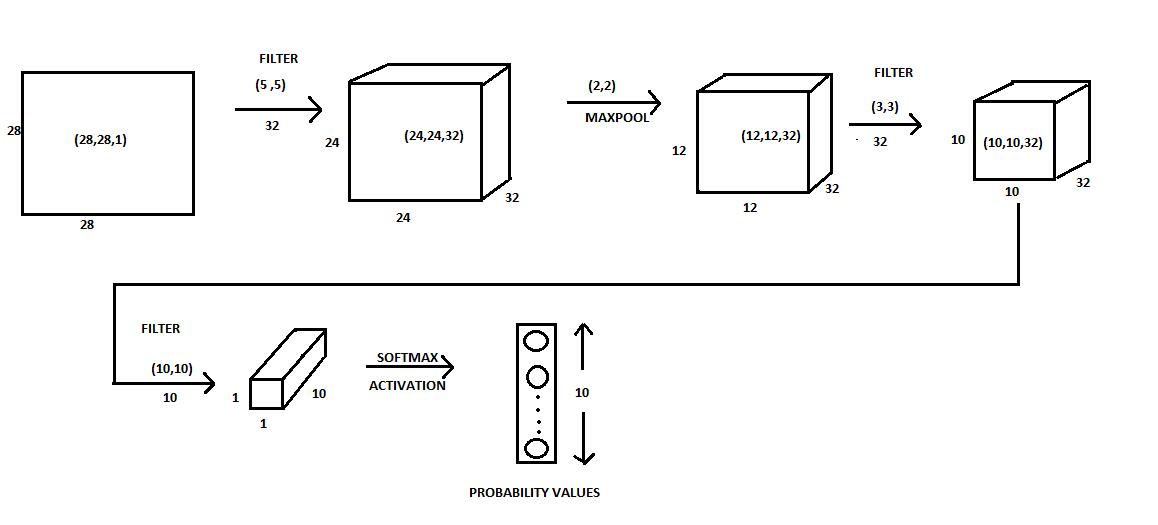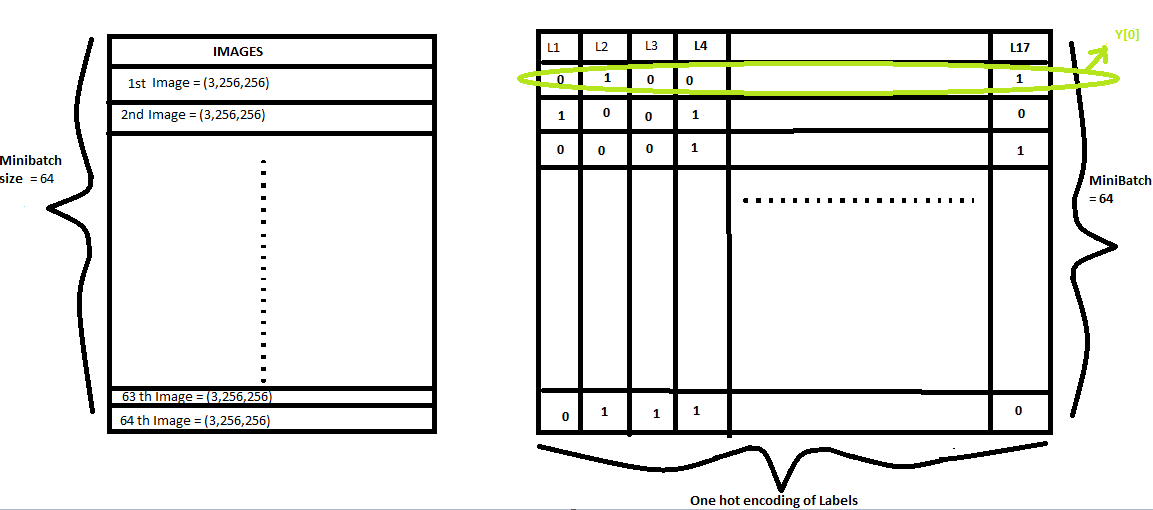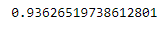# How to create a State of the Art Multi-Label Image Classifier?

Welcome to the Third Episode of Fastdotai where we will take on the Case of Muti-Label Classification. Before we start , I would like to thank Jeremy Howard and Rachel Thomas for their efforts to democratize AI.

To make best out of this blog post Series , feel free to explore the first Part of this Series in the following order:-

For those who haven’t seen previous episodes , please click here to check out 2.1 , 2.2 .

Today we will deal with Multi-Label Classification, where we have more than one labels as target variable. Before going deep into Multi-Label Classification , let’s understand:-

HOW DOES A CNN (CONVOLUTIONAL NEURAL NETWORK) WORK?

There are various parts to the CNN architecture . Lets discuss them in detail , post which , we will combine them and discuss about CNN architecture in detail. So lets start with the input i.e Image

1. IMAGE:-

Initially, we have an image. An image is actually a grid of numbers. Looks something like this image:-This is how a gray-scale image representing # 7 looks like . The pixel values has been standardized between 0 and 1.

2. KERNEL/FILTER:-

On top of the image we have a kernel . A kernel / filter, in this case, is a 3 by 3 slice of a 3d tensor that helps us to perform convolution.

This 3 by 3 slice kernel slides over the image and give rise to feature maps .

3. ACTIVATION:-

A feature map is made up of activations. An activation is a number which is calculated by

• Taking a slice of input of the same dimension .
• Make sure your kernel is of the same dimension as that of the slice of input.
• Perform an element-wise multiplication of the input we got from Step 1 with the kernel we have from Step 2
• Then sum it up.
• It gives rise to a number say ‘N’ .
• Apply ReLu(Rectified Linear Unit) activation function on top of that. Basically ReLu means `max(0,N).`
• The number that we get is known as ‘Activation’.

4. TENSOR:-

Assume that our network is trained and at the end of training it has created a Convolutional filter with the kernels values that have learned to recognize vertical and horizontal edges. Pytorch doesn’t save these filters values as two different 9 digit arrays. It stores the values as tensor. A tensor is a high dimensional array. A Tensor has an additional axis which helps us to stack each of this filters together.

5. HIDDEN LAYER:-

All layer except the input layer and the output layer is known as the hidden layer . The layer that makes up the activation map is one such hidden layer. Its generally named as Conv1 and Conv2 and are the results of convolution of kernels.

Then we have got a non-overlapping 2 by 2 Maxpooling . It halves the resolution by height and width. Generally, its named as Maxpool .

On top of that, we have got dense layers /fully connected layers . For every single activation present in max-pool layer we create a weight corresponding to that which is known as the fully connected layer. Then do a sum product of every single activation with every single weight. This will give rise to a single number.

Cons of using extra Fully Connected Layer :- It leads to overfitting and also slow processing.

Note:- The dimension of the kernel and the dimension of the slice of image/activation map should always be the same. For Multi-channel input make multi-channel kernels. This helps in higher dimension linear combination.

HOW THE KERNEL VALUES GETS UPDATED?

Basically, we start with some random kernel values and then use stochastic gradient descent to update the kernel values during training so as to make sense of the values in the kernel . In this way after a couple of epochs, we reach to a position where initial layer kernels are detecting edges, corners and subsequently higher layer kernels are learning to recognize more important feature.

MY TAKE ON SIMPLE CNN USING KERAS TO CLASSIFY MNIST DATASET

SIMPLIFIED DIAGRAM REPRESENTING THE CODE (CNN IN A NUTSHELL)CNN in detail

So we started out with a (28,28,1) input Image.

1. We used filters/kernels to reduce or increase the depth/breadth of the Activation map and decrease the height and width of the Activation map. When we convolve an input image of dimension (28,28,1) with 32 numbers of kernel having the dimension (5,5) we get an output of (24,24,32).
2. The output dimension is calculated using {(n-f+1) ,(n-f+1) ,(#Kernels)} where
• n= Image dimension
• f=kernel dimension
• #Kernels=Number of Kernels
• So we get {(28–3+1) ,(28–3+1) ,(#Kernels)}=(24,24,32)

3. We use Non-Linear Function/ReLU activation function in deep learning . But why? Check out this Quora post below.

Why does deep learning/architectures only use the non-linear activation function in the hidden…
Answer (1 of 9): “Why is a nonlinear activation function used?” Without a nonlinear activation function, the neural…www.quora.com

4. Post that we used max-pooling to reduce the height and width of the kernel by a factor of 2.

• Hence the activations map (24,24,32) is reduced to (12,12,32).

5. This (12,12,32) activation map is convolved with 32 numbers of kernel having dimension (3,3) and the output dimension now as per the formulae {(n-f+1),(n-f+1), (#Kernels)}={(12–3+1),(12–3+1),32}=(10,10,32) .

6. This (10,10,32) activation map is convolved with 10 number of kernels having a dimension (10,10) and the output dimension now as per the formulae

{(n-f+1),(n-f+1),(#Kernels)}={(10–10+1),(10–10+1), 10}=(1,1,10).

7. Finally, we have reached to a point where we have (1,1,10) dimension of activation . Its the penultimate layer . It spits out 10 random numbers.

8. We then use softmax activation on top of that to convert the numbers into probabilities.

9. Softmax Activation returns probability values for 10 numbers ranging from (0,1,2,3,….9) and it also tend to pick up one thing particularly strongly. Softmax only occurs in the final layer. These will be our predicted values. As these are probability values so it adds up to result 1. We will compare these predicted values with our target values . Please check line #9 of the above attached code(keras.ipynb) to know how the target values are saved in One hot encoded form. The number 5 is represented as below.

10. After that, we will try to minimize the loss between 10 predicted values and the 10 target values using Loss function. To compute the loss function we use the concept of Gradient Descent. Using Gradient Descent keep updating the parameters/kernel values.

11. Finally, consider the parameters corresponding to the point of minimum loss. And use those parameters/kernel values during prediction on the test dataset. This is the concept for Single label classification like dogs vs cats or dog breed classification. Now let’s see a case of Multi-Label Classification.

A best example of Multi-Label Classification is the kaggle competition Planet: Understanding the Amazon from Space . So let’s do it in couple of steps

1.DOWNLOAD THE DATA AND IMPORT THE PACKAGES REQUIRED .

Use the below command to Download the data

`! pip install kaggle import kaggle! kaggle competitions download -c planet-understanding-the-amazon-from-space!pip install fastai==0.7.0!pip install torchtext==0.2.3`
`!pip3 install http://download.pytorch.org/whl/cu80/torch-0.3.0.post4-cp36-cp36m-linux_x86_64.whl !pip3 install torchvision`

Import the Packages and check whether the files are present in the directory

`train_v2.csv `file has the names of the files that are present in the training dataset and the `labels `corresponding to them.

2. GET FAMILIAR WITH DATA USING PANDAS

DIFFERENCE BETWEEN SINGLE AND MULTI-LABEL CLASSIFICATION

In a Single label classification, the image is either a cat or a dog like the one below:-

Lets check for the Multi-label Classification :-

As we can see the output , in the case of Multi-Label classification, images are classified into two parts

• Weather — There are many types of weather mentioned in the data. Out of which we can see the haze and clear in the above snapshot.
• Features —List of features in the images above is primary , agriculture, water .

Primary stands for the primary rain forest .

Agriculture stands for a cleared area used for agricultural land.

Water stands for the river or lake.

Basically, in multi-label classification, each image belongs to one or more classes. In the example shown above the 1st image belongs to two classes: haze and primary rainforest . The 2nd image belongs to 4 classes: Primary, clear, Agriculture and Water. Softmax isn’t a good activation function to classify these images , as it has a tendency to classify an image into 1 category strongly and not multiple categories. Hence Softmax is good for Single Label Classification and not good for Multi-Label Classification.

Fastai looks for the labels in the `train_v2.csv `file and if it finds more than 1 label for any sample, it automatically switches to Multi-Label mode.

As discussed in Episode 2.2 , we create a validation dataset which is 20% of the training dataset . The below mentioned commands are used for the creation of validation dataset:-

3. GET DATA IN FASTAI FORMAT

These steps are the same as we did in the previous two episodes. `get_data(sz)` has two lines of code:-

• The tfms_from_model helps in data augmentation. The `aug_tfms=transforms_up_down` which means to flip the images vertically . It’s actually doing more than that. There is actually 8 possible symmetry to a square image which means it can be rotated through 0,90,180,270 degrees and for each of them it can be flipped . That’s a complete enumeration of everything we can do to symmetries of a square . Its known as `dihedral `group. This code will do full 8 set of flips i.e the `dihedral `set of rotations and flips plus small 10 degree rotations ,a little bit of zooming , a little bit of contrast and brightness adjustment. To know more about this please check `transforms.py` file within the `fastai `folder. A snippet from `transforms.py` that performs dihedral rotation has been attached below. Please check what else transformations we apply in `transforms.py` file.
• PARAMETERS FOR `ImageClassfierData.from_csv(...)` ARE:

It helps in reading the files as per fastai format.

• `PATH`is a root path of the data (used for storing trained models, precomputed values, etc) .Also contains all of the data.
• `'train'` — the folder that contains the training data.
• The `labels.csv` file has the labels for planet images.
• `val_idxs `has the validation data . It indicates the index number in labels.csv that has been put into the validation dataset .
• `test_name='test' `is the `test `dataset.
• The file names actually have a `.jpg` at the end which is not mentioned in the `labels.csv` file hence we have `suffix=’.jpg’` . This will add `.jpg` to the end of file names.
• `tfms `is the transformation we are going to apply for data augmentation.
• `bs`= batch_size of 64 images.

The Concept of Data Loader vs Dataset in Pytorch :-

A dataset which we came across in previous episodes, will return a single image and a data loader will return a mini batch of images. We can get only the next mini batch . To turn a data loader into an iterator we used a standard python function known as iter. That’s an iterator . To fetch the next minibatch pass the iter to next. It will return the next minibatch of images and the labels. This has been described below:-

`x,y = next(iter(data.val_dl))y`

The above command is a validation set data loader and will return a minibatch of images and labels. The `y` label gives the output below

As we can see there are 17 labels in this minibatch of 64 samples. The `bs=64 `has been explicitly mentioned in the `get_data(bs)` function above. To make sense of what does these one hot encoded label means check out the code below:-

`list(zip(data.classes, y))`

The data.classes has the actual label names and `y` gives the name of all the labels that particular sample belongs to .The output ,as shown above, represents that the 1st image has the labels agriculture, clear, primary and water . The one hot encoding representation of the labels has been represented in the below image. The one-hot encoding of Labels is internally handled by the Pytorch framework.Data Representation

This one hot encoded representation of the labels are the Actual values . The Neural Network spits 17 such values (in this case ) which are known as the Predicted values. We use the Concept of Loss function and Gradient Descent to minimize the error between the actual and predicted values .

In some cases, the image isn’t that clear . In such scenarios to get a hang of what all feature the image has, increase the brightness of the image by using a multiplication factor of 1.5/1.6/1.7 as shown below.

The best part of working with this Planet data is that it’s not similar to ImageNet . While working with data in real-world scenario, we don’t have the data similar to ImageNet dataset.

Here we start out by resizing data to (64,64) instead of it’s original size (256,256) . We wouldn’t start such small in case of Dogs vs Cats Classification as the pretrained resnet network starts off nearly perfect, so if we resize everything to (64,64) and retrain the weights, it will destroy the weights that were earlier pretrained to be good. Most ImageNet models are trained on top of (224,224) or (299,299). The main reason we are starting so small is that ImageNet images weren’t similar to this Planet Competition dataset. The main takeaway from the Resnet network that has been trained on ImageNet dataset are the initial layers which can detect edges , corners ,textures and repeating patterns .

Here is how it works:-https://machinelearningmarvel.in/media/6ec7d531efb51d4199420884f594325f

Get the data of the required size i.e (64,64).

`sz=64data = get_data(sz)data = data.resize(int(sz*1.3), '/tmp')`

4. SETUP THE NEURAL NETWORK AND LOOK FOR THE BEST LEARNING RATE

`from planet import f2`
`metrics=[f2]f_model = resnet34`
`learn = ConvLearner.pretrained(f_model, data, metrics=metrics)`

The f2 metrics has been discussed later on in this blog post. Furthermore in this model since it’s not mentioned `precompute=True` , hence by default it takes `precompute=False`. To know this click `shift+Tab` , and it will display all the parameters with their default values. At this point in time when `precompute=False `,

• Our Data Augmentation is ON.
• All layers up to the penultimate layer are Frozen.
• After the penultimate layer , we have got Extra Fully Connected Layer attached to the end and then we have our final output.

Now, let’s look out for the best learning rate finder.

`lrf=learn.lr_find()learn.sched.plot()`

As we see in the Loss vs Learning Rate Graph , the best learning can rate is somewhere near 0.2. How ?

• As discussed earlier the loss is minimum at 0.2 on the Y axis and the learning rate corresponding to this is 10⁰ =1 on the x axis .
• As discussed earlier ,we can get the best learning rate just before the point where the loss is minimum , hence it’s 0.2.

Now using the best learning rate `0.2` , lets train our model as shown in the below code.

5. TRAIN LAST LAYER FROM PRECOMPUTED ACTIVATIONS FOR COUPLE OF EPOCHS.

`lr = 0.2learn.fit(lr, 3, cycle_len=1, cycle_mult=2)`

The concept of `Cycle_len `and `Cycle_mult `has been discussed in detail in Episode 2.1 . Until now we are training only the extra fully layers that we have connected at the end.

To train all the layers until the end ,

• Set differential learning rate for subsets of layers.
• Unfreeze the frozen layers .
• Start training all the layers.

To learn a different set of features or to tell the learner that the convolution filters are needed to be changed , simply `unfreeze `all the layers .A frozen layer is one whose weights are not trained or updated. To know how unfreezing and freezing of layers work check out Episode 2.1 . Since the images in Planet competition are not like ImageNet Dataset :-

• So the learning rate is high.
• And earlier layers need to learn more than the later layers.

6.UNFREEZE THE LAYERS AND TRAIN ALL OF THE LAYERS .

`lrs = np.array([lr/9,lr/3,lr])learn.unfreeze()learn.fit(lrs, 3, cycle_len=1, cycle_mult=2)`

Below we can see the major drop in Loss, after each cycle .

`learn.save(f’{sz}’)learn.sched.plot_loss()`

7. IMPROVE OUR MODEL AND KEEP OVERFITTING AT BAY :-

From now onwards we will be increasing our image size to (128,128) and further to (256,256) so as to

• Reduce Overfitting .
• Decrease the gap between trn_loss and val_loss .
• Train the earlier layer (by unfreezing them) of the Neural Network as the pre-trained weights are from ImageNet model which doesn’t share much resemblance with Planet Competition dataset.
`sz=128learn.set_data(get_data(sz))learn.freeze()learn.fit(lr, 3, cycle_len=1, cycle_mult=2)`
`learn.unfreeze()learn.fit(lrs, 3, cycle_len=1, cycle_mult=2)learn.save(f'{sz}')`
`sz=256learn.set_data(get_data(sz))learn.freeze()learn.fit(lr, 3, cycle_len=1, cycle_mult=2)`
`learn.unfreeze()learn.fit(lrs, 3, cycle_len=1, cycle_mult=2)learn.save(f'{sz}')`

Finally we do a TTA(Test Time Augmentation) to get the .

`multi_preds, y = learn.TTA()preds = np.mean(multi_preds, 0)f2(preds,y)`Accuracy

Voilaaaaaaa , we get an accuracy of `93.6%` which is too good for Multi-label Classification.

If you are with me until this point , give yourself a high-five.

?????????? ANY QUESTIONS, PLEASE RAISE YOUR HANDS ??????????

Qs 1:- What does `data.resize() `do in the command below?

`data = data.resize(int(sz*1.3), '/tmp')`

If the initial input is (1000,1000) reading that jpeg and resizing it to( 64,64) turns out to take more time than training the convnet does for each batch . What resize does is it says that it’s not gonna use any image bigger than sz*1.3. So go through ones and create new jpegs of `size=sz*1.3` . This step is not necessary but it speeds up the process.

Qs 2:- Why metrics used here in Planet Satellite Image Competition is `f2 `instead of accuracy ?

There are a lot of ways to turn the confusion matrix that we saw in dog vs cat classification into an accuracy score .Those are

• Precision
• Recall
• f-Beta

As per this competition criteria, the accuracy is judged on the basis of f-Beta score .In the f-Beta score , Beta says how much you weight false negatives vs false positives ? Here in f-Beta , Beta value is 2. We are passing this as a `metrics `when we are setting up the Neural Network. Check out the code below.

`from planet import f2`
`metrics=[f2]f_model = resnet34`
`learn = ConvLearner.pretrained(f_model, data, metrics=metrics)`

Qs 3:- Difference between Multi-Label and Single-Label Classification?

The output activation function for a single label classification problem is Softmax . But in case we have to predict multiple labels in a particular image as shown below in the last column:-

Then Softmax is a terrible choice as it has a tendency to pick up a particular label strongly. For the multi-label classification problem, the activation function we use is Sigmoid . The fastai library automatically switches to Sigmoid if it observes a multi-label classification problem. Sigmoid formula is e^x/(1+e^x) . And Sigmoid graph looks like :-

Basically what Sigmoid graph signifies is , if activation is less than 0.5 then Sigmoid will return a low probability value and if the activation >0.5 the Sigmoid will return a high probability value. That’s how multiple things can have high probabilities.

Qs 4:- How training of layers work?

The layers are very important but the pre-trained weights in them aren’t . So it’s the later layers that we want to train the most. The earlier layer already is closer to what we want i.e detecting edges and corners.

So in case of dogs vs cats , when we are creating a model from a pretrained model it returns a model where all the convolution layers are frozen and some randomly set fully connected layer that has been added to the end are unfrozen .So when we say fit, at first , it trains the randomly initialized fully connected layers at the end. If something is really close to Imagenet dataset , that’s often what we need . As the earlier layers are already good at finding edges , gradients , repeating patterns etc. Then when we unfreeze we set the learning rate for the earlier layers to be really low as we don’t want to change them much .

Whereas in the Satellite data , the earlier layers are like better than the later layers but we still need to change them quite a bit and that’s why our learning rate is just 9 times smaller than final learning rate rather than 1000 times smaller like in the previous case .

Qs 5:- Why can’t we directly start with unfreezing all the layer?

We can do that , but it’s going to take some more time . At first, by unfreezing the final layers and keeping the initial layers frozen, we are training the final layers to learn more important features . The convolutional layers contain pretrained weights so they are not random .For things that are close to ImageNet they are really good but if they aren’t close to ImageNet they are better than nothing .All of our FC layers are totally random , so therefore you would always want to make the fully Connected layers better than random by training them a bit first , because otherwise if you go straight to unfreeze , then we would be fiddling around the early layer weights when later ones are still random.

Couple of points to remember:-

• Training means updating weights of kernel values and weights of FC layer. Activation are calculated from weights and previous layer activation outputs.
• `learn.summary() `command is used to visualize the model.

If you like it , then ABC (Always be clapping . 👏 👏👏👏👏😃😃😃😃😃😃😃😃😃👏 👏👏👏👏👏)

If you have any questions, feel free to reach out on the fast.ai forums or on Twitter:@ashiskumarpanda

P.S. -This blog post will be updated and improved as I further continue with other lessons. In case you are interested for the source code check it out here .

To make best out of this blog post Series , feel free to explore the first Part of this Series in the following order:-

Edit 1:- TFW Jeremy Howard approves of your post . 💖💖 🙌🙌🙌 💖💖 .